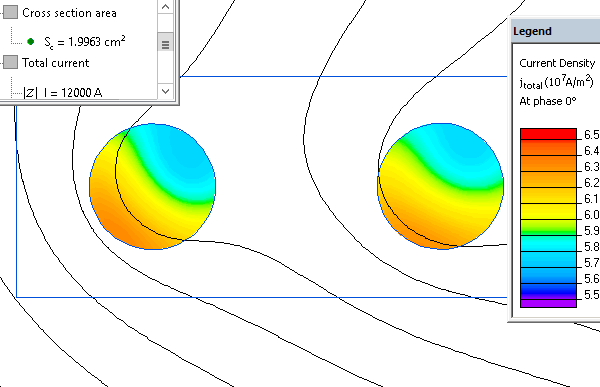Main >> Applications >> Sample problems

# High frequency line trap

air core coil, wave trap, transmission line filter, multiphysics analysis

An example of the calculation of the forces and mechanical stresses for the high-frequency line trap at the short circuit current.

Problem Type
Axisymmetric multiphysics problem of AC magnetics coupled to Stress analysis.

Geometry

Given
Current (peak value) I = 12 kA, frequency f = 50 Hz.
Aluminum cable:
cross-section area A = 2 cm².
conductivity γ= 37 MS/m;
Young's modulus E = 70 GPa;
Poisson's ratio ν = 0.34;

Fiberglass case:
Young's modulus E = 20 GPa;
Poisson's ratio ν = 0.11;

Determine forces and mechanical stresses in the high-frequency line trap body.

Solution
The actual spring-coil is modelled as a set of circular turns embedded in a fiberglass case. That facilitates 2D axisymmetric simulation.
The solution consists of two stages: the calculation of forces in a AC Magnetic problem, and then the calculation of mechanical stresses in a mechanical problem. The transfer of forces from AC Magnetic problem to mechanical one is automated using the coupling mechanism.

Results
Current density distribution in the aluminum cable:Mechanical stress in the aluminum cable, wave trap deformed shape (zoomed 1000:1) and Ampere force value acting on the ending turn:*Reference: Line trap in Wikipedia.

• Video: Line trap
•